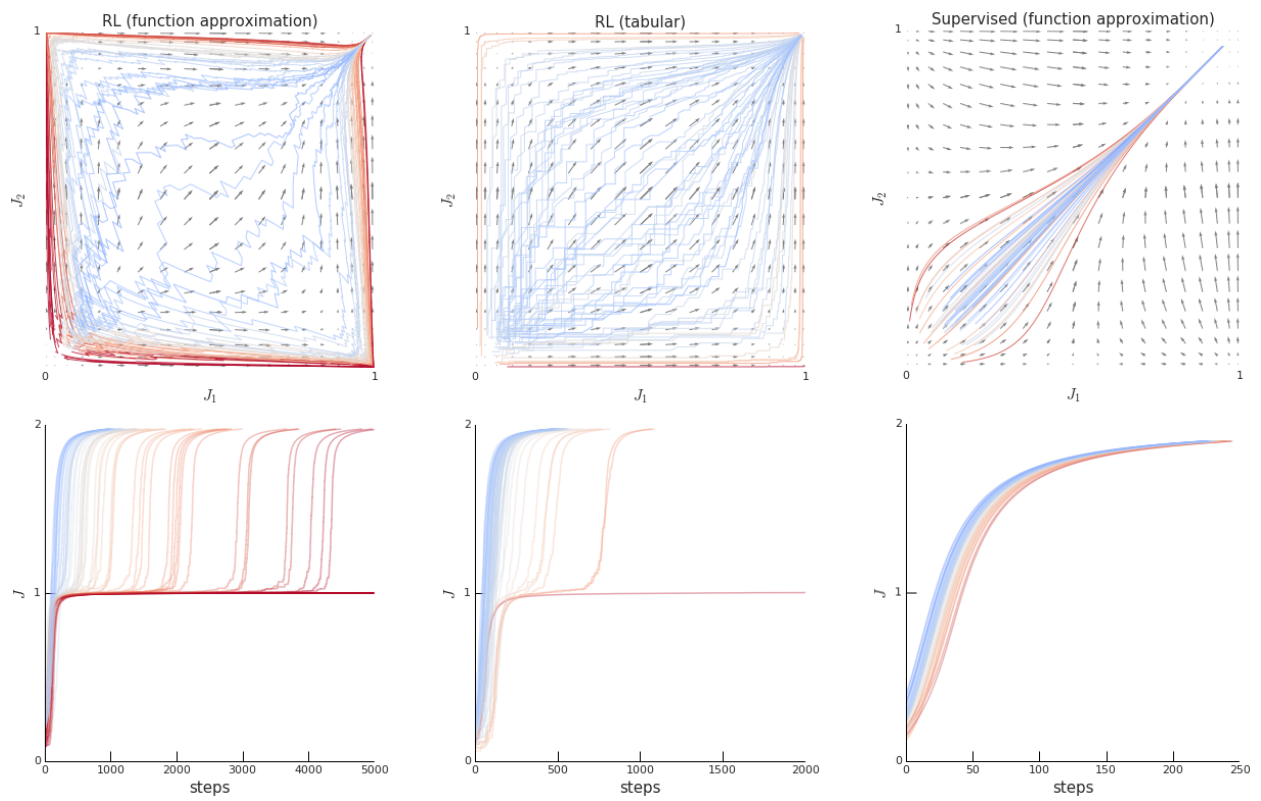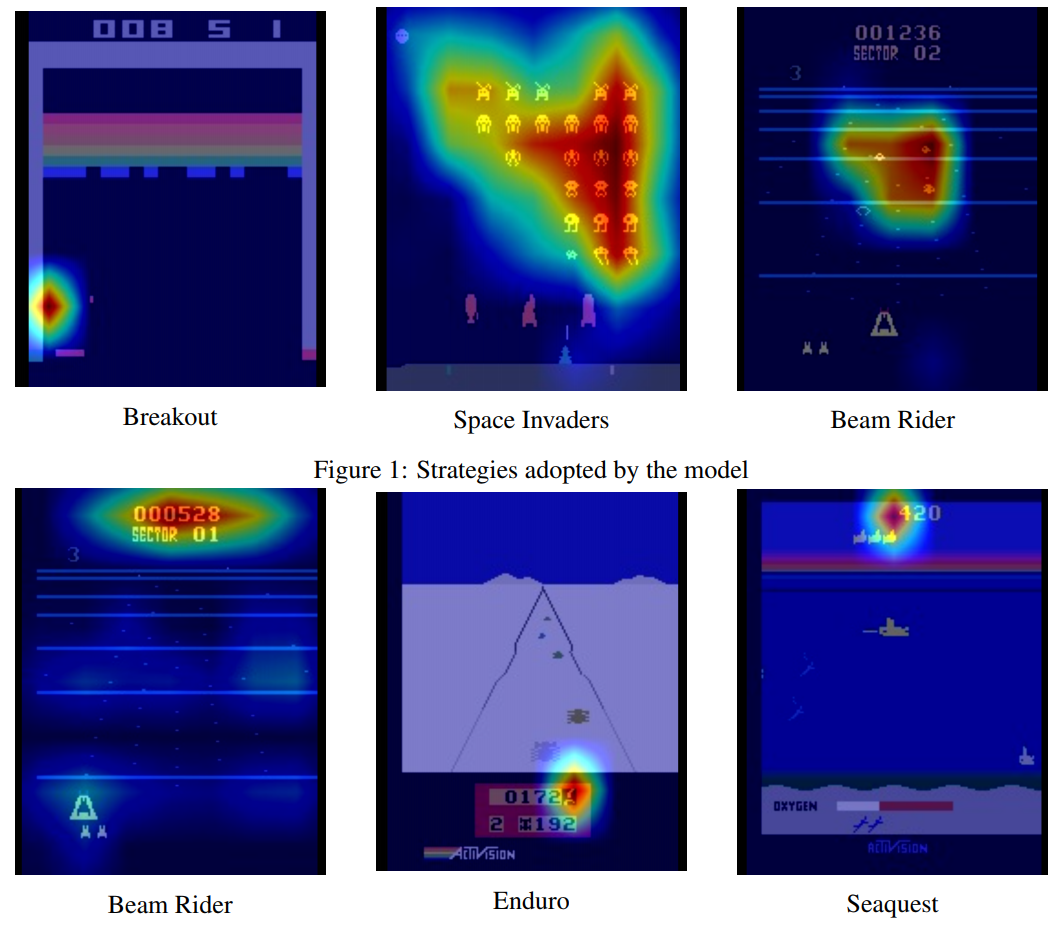## Ray Interference: Why performance plateaus might occurWhat it is

Researchers at Google DeepMind investigated a possible cause of performance plateaus. When the learning objective can be decomposed into multiple components, these components could negatively interfere with each other. In that case, improvement in one dominant component halts learning in other components until the dominant component is learned. For deep RL algorithms with coupled performance and learning progress (i.e. on-policy algorithms), this results in long plateaus for overall performance.

The left subplots in the figure above shows a minimal example of bandit with 2 objectives (J). Each colored line in the top plot is a sample trajectory, and the colors correspond to the learning curves in the bottom plot. Unlike the tabular case or supervised learning case, most trajectories for the function approximated case pass by the saddle points $(0, 1)$ and $(1, 0)$ and hit plateaus. (This phenomenon is coined “ray interference,” due to the ray-like shape of the top plot.) With more number of components, more plateaus appear, and later plateaus take exponentially longer time.

It is simple to detect ray interference if the components are known, since simply monitoring the progress of each component can show if some are stalling. However, in most cases these decompositions are implicit. In this case, the authors recommend checking the learning curves of individual runs (not averaged runs) for plateaus and running control experiments.

Why it matters

Although multi-component objective might not seem prevalent, many existing RL environment exhibit such property. For example, in domains where rewards are very sparse, each reward can be viewed as a distinct objective. As such, decompositions need not be explicit. The paper does not answer how prevalent this issue is in existing algorithms but conjectures that performance plateaus observed in Atari domains could be due to ray interference.

## Compression and Localization in RL for Atari GamesWhat it is

Researchers at Carnegie Mellon University compressed a DQN agent, reducing 1.68M parameters to 43.1K parameters while maintaining similar performance in 6 Atari games. The authors first train an “expert” DQN agent with the original architecture, then train a student network with reduced number of feature maps. The Q values of the student agent and the expert agent is converted to a probability distribution, and the student agent is trained to minimize the KL divergence of two distributions.

To further reduce the number of parameters, the student network also has a spatial max pooling layer after the convolutional layers. Apart from reducing the number of parameters, this also serves as an implicit form of attention, as the max-pooling layer forces the network to assign highest activations to most relevant entities. These max-pooled layers can also be upsampled and superimposed to the original frame to understand the agent better, as seen above.

Why it matters

In many real life applications, lightweight neural networks are required due to restrictions in space and time. However, it is difficult to train a small neural network from scratch with performance comparable to that of large neural networks. Thus, such distillation process is needed to train a small neural network with good performance.